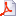##### Personal tools
You are here: Home Carrascal et al. (2009). Partial least squares regression as an alternative to current regression methods used in ecology. Oikos, 118: 681-690.

# Carrascal et al. (2009). Partial least squares regression as an alternative to current regression methods used in ecology. Oikos, 118: 681-690.

Behavioral ecologists must often face the problem of dealing with large sets of predictor variables in relation to sample size, especially in experimental studies where sample size is limited due to methodological difficulties. In addition, these predictor variables are often highly correlated among them. These problems have been traditionally solved by applying multiple regression (MR) after variable-selection or multivariate reduction methods, or by performing best-subsets techniques. However, removing some variables prior to the analysis may lead to the loss of some variability that, albeit less important than other variables, could still be biologically significant. Multivariate reduction methods also present problems, as they reduce the initial variability in the predictor variables independently of their covariation with the response variable. Lastly, best-subsets techniques may conclude that several models are equally probable. The partial least squares regression analysis (PLSR) is an alternative to these methods because: 1) PLSR models explain a similar amount of variance to results obtained with MR and with MR after principal components analysis (a multivariate reduction method), and 2) PLSR is more reliable than other techniques when identifying relevant variables and their magnitudes of influence, especially in cases of small sample size and high correlation between predictor variables.Carrascal et al. 2009 - Oikos.pdf — PDF document, 239Kb

##### Document Actions